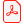# Konferensartikel

## Connected Minimal Acceleration Trigonometric Curves

Tony Barrera
Barrera Kristiansen AB

Anders Hast
Creative Media Lab, University of Gävle, Sweden

Ewert Bengtsson
Centre for Image Analysis, Uppsala University, Sweden

Ladda ner artikelhttp://www.ep.liu.se/ecp_article/index.en.aspx?issue=016;article=001

Linköping Electronic Conference Proceedings 16:1, s. 1-5

Visa mer +

ISBN:

ISSN: 1650-3686 (tryckt), 1650-3740 (online)

### Abstract

We present a technique that can be used to obtain a series of connected minimal bending trigonometric splines that will intersect any number of predefined points in space. The minimal bending property is obtained by a least square minimization of the acceleration. Each curve segment between two consecutive points will be a trigonometric Hermite spline obtained from a Fourier series and its four first terms. The proposed method can be used for a number of points and predefined tangents. The tangent length will then be optimized to yield a minimal bending curve. We also show how both the tangent direction and length can be optimized to give as smooth curves as possible. It is also possible to obtain a closed loop of minimal bending curves. These types of curves can be useful tools for 3D modelling; etc.

### Nyckelord

Trigonometric curves; Hermite curves; least square minimization

### Referenser

V. ALBA-FERNANDEZ; M.J. IBANEZ-PEREZ; M.D. JIMENEZGAMERO 2004. A Bootstrap Algorithm for the Two-Sample Problem Using Trigonometric Hermite Spline Interpolation Communications in Nonlinear Science and Numerical Simulation. Vol. 9. Num. 2. Pag. 275-286

T. BARRERA; A. HAST; E. BENGTSSON 2005. Minimal Acceleration Hermite Curves Graphics Programming Gems V; Charles River Media; Edited by Kim Pallister; pp 225-231.

R.H. BARTELS; J.C. BEATTY;AND B.A. BARSKY 1998. An Introduction to. Splines for use in Computer Graphics and. Geometric Modeling ”Hermite and Cubic Spline Interpolation.” Ch. 3; pp. 9-17.

R. L. BURDEN; J.D. FAIRES 1989. Numerical Analysis Numerical Analysis; PWS-KENT Publishing company Boston; pp. 439; 440.

E. CATMULL; R. ROM 1974. A Class of Local Interpolating Splines Computer Aided Geometric design; pp. 317-326.

A. FOLEY; J. D.; ET AL 1997. Computer Graphics: Principles and Practice; 2nd ed. Addison-Wesley; p. 480.

X. HAN 2003. Piecewise Quadratic Trigonometric Polynomial Curves Mathematics of Computation; pp. 1369-1377.

D. HEARN; M.P BAKER 2004. Computer Graphics with OpenGL Pearson Education Inc.; pp. 426-429.

E. LENGYEL. 2004. E. Lengyel; Mathematics for 3D Game Programming and Computer Graphics; 2nd ed. E. Lengyel; Charles River Media; pp. 433-436.

T. LYCHE. 1979. A Newton form for Trigonometric Hermite Interpolation

E. Lengyel; Charles River Media; pp. 433-436.

PRESS ET AL. 1992. Numerical Recipes in C. [Press92] Cambridge University Press; pp. 74-75.

I. J. SCHOENBERG 1964. On Trigonometric Spline Interpolation Journal of Mathematics and Mechanics; pp. 795-825.

A. VLACHOS; J ISIDORO Smooth C2 Quaternion-based Flythrough Paths Game Programming Gems 2; Charles River Media; Edited by Mark Deloura; pp. 220-227.

G. WALZ Identities for Trigonometric B-Splines with an Application to Curve Design Identities for trigonometric B-splines with an application to curve design. BIT 37; pp. 189-201.